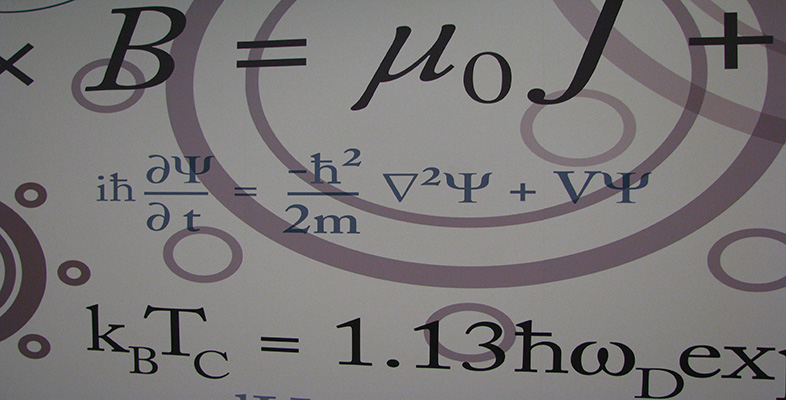Number systems

This free course is available to start right now. Review the full course description and key learning outcomes and create an account and enrol if you want a free statement of participation.

Free course

2.5 Modulus of a complex number

We also need the idea of the modulus of a complex number. Recall that the modulus of a real number x is defined by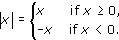For example, |7| = 7 and |−6| = 6.

In other words, |x| is the distance from the point x on the real line to the origin. We extend this definition to complex numbers as follows.

Definition

The modulus |z| of a complex number z is the distance from the point z in the complex plane to the origin.

Thus the modulus of the complex number z = x + iy is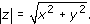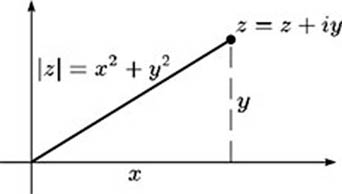For example, if z = 3 − 4i, then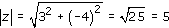.

Exercise 11

Determine the modulus of each of the following complex numbers.

• (a)  5 + 12i

• (b)  1 + i

• (c)  −5

Solution

• (a)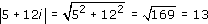• (b)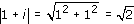• (c)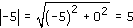The modulus of a complex number has many properties similar to those of the modulus of a real number.

Properties of modulus

1. |z| ≥ 0 for any z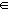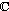, with equality only when z = 0.

2. |z1z2| = |z1||z2| for any z1, z2.

Property 1 is clear from the definition of |z|. Property 2 can be proved in a similar way to property 2 of complex conjugates given in Exercise 10.

The following useful result shows the link between modulus and distance in the complex plane.

Distance Formula

The distance between the points z1 and z2 in the complex plane is |z1z2|.

This is obtained by applying Pythagoras' Theorem to the triangle in the diagram below.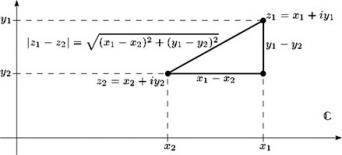Exercise 12

For each of the following pairs z1, z2 of complex numbers, draw a diagram showing z1 and z2 in the complex plane, and evaluate |z1z2|.

• (a)  z1 = 3 + i,   z2 = 1 + 2i.

• (b)  z1 = 1,   z2 = i.

• (c)  z1 = −5 − 3i,   z2 = 2 − 7i.

Solution

• (a)

•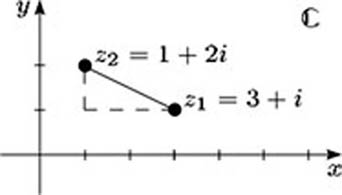• Here

•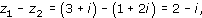• so

•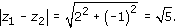• (b)

•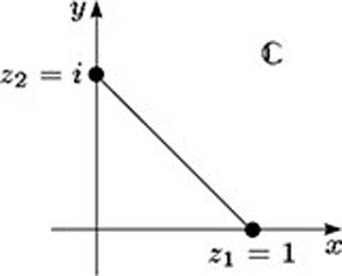• Here

•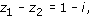• so

•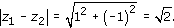• (c)

•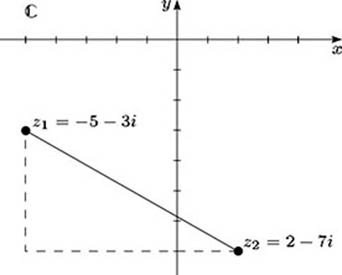• Here

•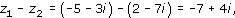• so

•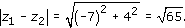The following properties describe the relationship between the modulus and the complex conjugate of a complex number.

Conjugate–modulus properties

1.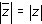for all z.

2.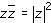for all z.

To prove these properties, we let z = x + iy. Then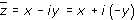,

so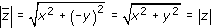and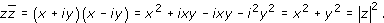M208_6